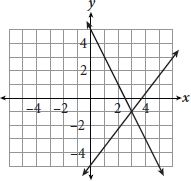# SAT Math Multiple Choice Question 797: Answer and Explanation

### Test Information

Question: 797

2.The graph of a system of linear equations is shown above. If the ordered pair (x, y) represents the solution to this system, what is the value of xy?

• A. –4
• B. –2
• C. 2
• D. 4